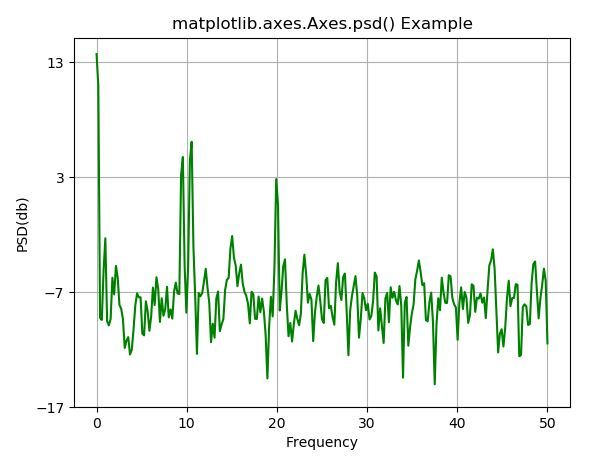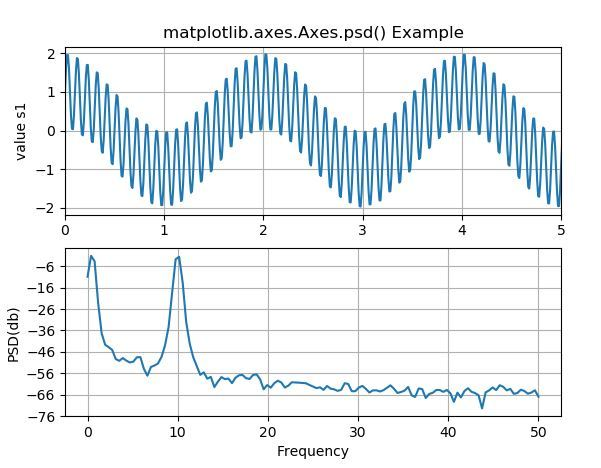Related Articles
Matplotlib.axes.Axes.psd() in Python
• Last Updated : 13 Apr, 2020

Matplotlib is a library in Python and it is numerical – mathematical extension for NumPy library. The Axes Class contains most of the figure elements: Axis, Tick, Line2D, Text, Polygon, etc., and sets the coordinate system. And the instances of Axes supports callbacks through a callbacks attribute.

## matplotlib.axes.Axes.psd() Function

The Axes.psd() function in axes module of matplotlib library is used to plot the power spectral density.

Syntax: Axes.psd(self, x, NFFT=None, Fs=None, Fc=None, detrend=None, window=None, noverlap=None, pad_to=None, sides=None, scale_by_freq=None, return_line=None, *, data=None, **kwargs)

Parameters: This method accept the following parameters that are described below:

• x: This parameter is a sequence of data.
• Fs : This parameter is a scalar. Its default value is 2.
• window: This parameter take a data segment as an argument and return the windowed version of the segment. Its default value is window_hanning()
• sides: This parameter specifies which sides of the spectrum to return. This can have following values : ‘default’, ‘onesided’ and ‘twosided’.
• pad_to : This parameter contains the integer value to which the data segment is padded.
• NFFT : This parameter contains the number of data points used in each block for the FFT.
• detrend : This parameter contains the function applied to each segment before fft-ing, designed to remove the mean or linear trend {‘none’, ‘mean’, ‘linear’}.
• scale_by_freq : This parameter is allows for integration over the returned frequency values.
• noverlap : This parameter is the number of points of overlap between blocks.
• Fc : This parameter is the center frequency of x.
• return_line : This parameter include the line object plotted in the returned values.

Returns: This returns the following:

• Pxx:This returns the values for the power spectrum P_{xx} before scaling.
• freqs :This returns the frequencies for the elements in Pxx.
• line :This returns the line created by this function.

The resultant is (Pxx, freqs, line)

Below examples illustrate the matplotlib.axes.Axes.csd() function in matplotlib.axes:

Example-1:

 `# Implementation of matplotlib function ` `import` `numpy as np ` `import` `matplotlib.pyplot as plt ` `  `  `dt ``=` `0.01` `t ``=` `np.arange(``0``, ``30``, dt) ` `nse1 ``=` `np.random.randn(``len``(t)) ` `  `  `s1 ``=` `1.5` `*` `np.sin(``2` `*` `np.pi ``*` `10` `*` `t) ``+` `nse1 ``+` `np.cos(np.pi ``*` `t) ` `  `  `fig, ax1 ``=` `plt.subplots() ` `ax1.psd(s1``*``*``2``, ``512``, ``1.``/``dt, color ``=``"green"``) ` `ax1.set_xlabel(``'Frequency'``) ` `ax1.set_ylabel(``'PSD(db)'``) ` `  `  `ax1.set_title(``'matplotlib.axes.Axes.psd() Example'``) ` `plt.show() `

Output:Example-2:

 `# Implementation of matplotlib function ` `import` `numpy as np ` `import` `matplotlib.pyplot as plt ` `  `  `dt ``=` `0.01` `t ``=` `np.arange(``0``, ``30``, dt) ` `nse1 ``=` `np.random.randn(``len``(t)) ` `r ``=` `np.exp(``-``t ``/` `0.05``) ` `  `  `cnse1 ``=` `np.convolve(nse1, r, mode ``=``'same'``)``*``dt ` `  `  `s1 ``=` `np.cos(np.pi ``*` `t) ``+` `cnse1 ``+` `np.sin(``2` `*` `np.pi ``*` `10` `*` `t)  ` `  `  `fig, [ax1, ax2] ``=` `plt.subplots(``2``, ``1``) ` `ax1.plot(t, s1) ` `ax1.set_xlim(``0``, ``5``) ` `ax1.set_ylabel(``'value s1'``) ` `ax1.grid(``True``) ` `  `  `ax2.psd(s1, ``256``, ``1.``/``dt) ` `ax2.set_ylabel(``'PSD(db)'``) ` `ax2.set_xlabel(``'Frequency'``) ` `  `  `ax1.set_title(``'matplotlib.axes.Axes.psd() Example'``) ` `plt.show() `

Output:Attention geek! Strengthen your foundations with the Python Programming Foundation Course and learn the basics.

To begin with, your interview preparations Enhance your Data Structures concepts with the Python DS Course.

My Personal Notes arrow_drop_up
Recommended Articles
Page :